# ISEE Middle Level Quantitative : How to find mode

## Example Questions

### Example Question #1 : How To Find Mode

Kali decided to keep a log of how much money she spent on lunch at school each day for three weeks.  Her data included the following numbers:

Week 1: $2.50,$3.00, $2.75,$3.00, $2.50 Week 2:$3.00, $2.50,$2.75, $2.50,$3.00

Week 3: $2.50,$2.75, $2.50,$3.00, $2.50 What is the mode of this data? Possible Answers:$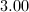$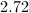$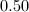$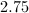$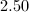$Explanation: In order to find mode, we must determine which number occurs most often in the data set. In this particular set,$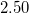occurstimes, $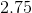occurstimes, and$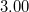occurstimes.  Since \$occurs the most often, it is the mode for this set of data.

### Example Question #2 : How To Find Mode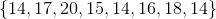Which measure of central tendency would you use to find the term that is most occuring?

Mode

Interquartile Range

Range

Mean

Median

Mode

Explanation:

Mode is the number that occurs most often, so that is the correct answer. Median is the middle number, while mean is the average of the set. Range is the difference between the greatest and least numbers.

### Example Question #3 : How To Find Mode

Give the mode of the data set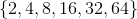The data set has no mode.The data set has no mode.

Explanation:

No element in the data set appears more than once; for an element to have a mode, that element must appear at least twice. The set has no mode.

### Example Question #271 : Isee Middle Level (Grades 7 8) Quantitative Reasoning

Which is the greater quantity?

(A) The mode of the data set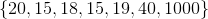(B) The median of the data set(A) and (B) are equal

(A) is greater

It is impossible to determine which is greater from the information given

(B) is greater

(B) is greater

Explanation:

Arrange the elements in ascending order.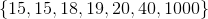The mode of the set is the element that appears most frequently, which here is 15.

The median of the set is the element that appears in the middle, which here is 19.

(B) is greater.

### Example Question #3 : How To Find Mode

Which is the greater quantity?

(A) The number of modes of the data set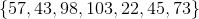(B)It is impossible to determine which is greater from the information given

(B) is greater

(A) and (B) are equal

(A) is greater

(B) is greater

Explanation:

The mode of a data set is the element that appears most frequently. The given data set has no repeated elements; it is considered to have no modes. (B) is greater.

### Example Question #1 : Mode

Consider the data set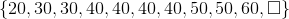Which of the following elements replaces the box to make 30 the mode of the data set?None of the other responses is correct.

None of the other responses is correct.

Explanation:

Regardless of what value replaces the box, 40 will still be the most frequently appearing element in the set (four times, more than any other element) - that is, 40 will be the mode.

### Example Question #272 : Isee Middle Level (Grades 7 8) Quantitative Reasoning

Consider the data setWhich of the following elements replaces the box to make 40 the mode of the data set?

(A)(B)(C)Only (A) is correct.

None of (A), (B), or (C) are correct.

Only (C) is correct.

Only (B) is correct.

(A), (B), and (C) are all correct.

(A), (B), and (C) are all correct.

Explanation:

Regardless of what value replaces the box, 40 will still be the most frequently appearing element in the set (four times, more than any other element). By definition, that makes 40 the mode.

### Example Question #4 : How To Find Mode

Which is the greater quantity?

(A) The number of modes of the data set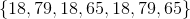(B)It is impossible to determine which is greater from the information given

(A) and (B) are equal

(A) is greater

(B) is greater

(B) is greater

Explanation:

Arrange the data set in ascending order.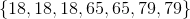18 appears three times; 65 and 79 appear two times each; therefore, 18 is the only mode, and (B) is greater.

### All ISEE Middle Level Quantitative Resources